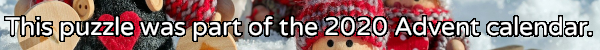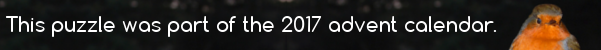mscroggs.co.uk
mscroggs.co.uksubscribe

# Puzzles

## 15 DecemberWhen talking to someone about this Advent calendar, you told them that the combination of XMAS and MATHS is GREAT. They were American, so asked you if the combination of XMAS and MATH is great; you said SURE. You asked them their name; they said SAM.
Each of the letters E, X, M, A, T, H, S, R, U, and G stands for a different digit 0 to 9. The following sums are correct:
Today's number is SAM. To help you get started, the letter T represents 4.

## 20 DecemberThe integers from 2 to 14 (including 2 and 14) are written on 13 cards (one number per card). You and a friend take it in turns to take one of the numbers.
When you have both taken five numbers, you notice that the product of the numbers you have collected is equal to the product of the numbers that your friend has collected. What is the product of the numbers on the three cards that neither of you has taken?

## 15 DecemberThere are 5 ways to make 30 by multiplying positive integers (including the trivial way):
• 30
• 2×15
• 3×10
• 5×6
• 2×3×5
Today's number is the number of ways of making 30030 by multiplying.

## Not Roman numerals

The letters $$I$$, $$V$$ and $$X$$ each represent a different digit from 1 to 9. If
$$VI\times X=VVV,$$
what are $$I$$, $$V$$ and $$X$$?

## Backwards fours

If A, B, C, D and E are all unique digits, what values would work with the following equation?
$$ABCCDE\times 4 = EDCCBA$$

## 10 DecemberHow many zeros does 1000! (ie 1000 × 999 × 998 × ... × 1) end with?

## 17 DecemberIn March, I posted the puzzle One Hundred Factorial, which asked how many zeros 100! ends with.
What is the smallest number, n, such that n! ends with 50 zeros?

## One hundred factorial

How many zeros does $$100!$$ end with?

## Archive

Show me a random puzzle
▼ show ▼
© Matthew Scroggs 2012–2021